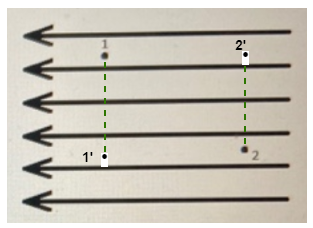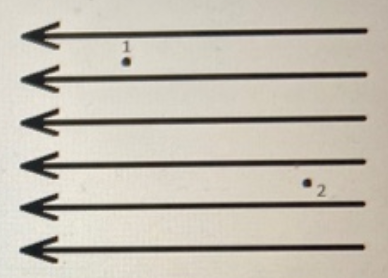# Problem: Two points, point 1 and point 2, are located inside a region with an electric field pointing to the left, as shown in the figure. Part (a) If a proton is moved from point 1 to point 2, how will the potential energy of the charge-field system change? How will the potential change?Part (b) If, instead, an electron is moved from point 1 to point 2, how will the potential energy of the charge-field system change? How will the potential change?

###### FREE Expert Solution

Potential energy:

$\overline{){\mathbf{U}}{\mathbf{=}}{\mathbf{q}}{\mathbf{∆}}{\mathbf{V}}}$

Let's consider the diagram:Part (a)

Points 2 and 2' are at equal potential since they lie on an equipotential surface.

The potential difference between two points is given by:

$\begin{array}{rcl}\mathbf{∆}\mathbf{V}\mathbf{\left(}\mathbf{r}\mathbf{\right)}& \mathbf{=}& \mathbf{-}{\mathbf{\int }}_{{\mathbf{r}}_{\mathbf{1}}}^{{\mathbf{r}}_{\mathbf{2}}}\mathbf{E}\mathbf{·}\mathbf{d}\mathbf{r}\\ & \mathbf{=}& \mathbf{-}{\mathbf{\int }}_{{\mathbf{r}}_{\mathbf{1}}}^{{\mathbf{r}}_{\mathbf{2}}}\mathbf{E}\mathbf{·}\mathbf{d}\mathbf{r}\mathbf{c}\mathbf{o}\mathbf{s}\mathbf{\left(}\mathbf{180}\mathbf{°}\mathbf{\right)}\\ & \mathbf{=}& \mathbf{E}{\mathbf{\int }}_{{\mathbf{r}}_{\mathbf{1}}}^{{\mathbf{r}}_{\mathbf{2}}}\mathbf{d}\mathbf{r}\\ & \mathbf{=}& \mathbf{E}\mathbf{\left(}{\mathbf{r}}_{\mathbf{2}}\mathbf{-}{\mathbf{r}}_{\mathbf{1}}\mathbf{\right)}\end{array}$

This shows that the potential is increasing.

99% (362 ratings)###### Problem Details

Two points, point 1 and point 2, are located inside a region with an electric field pointing to the left, as shown in the figure.Part (a) If a proton is moved from point 1 to point 2, how will the potential energy of the charge-field system change? How will the potential change?

Part (b) If, instead, an electron is moved from point 1 to point 2, how will the potential energy of the charge-field system change? How will the potential change?